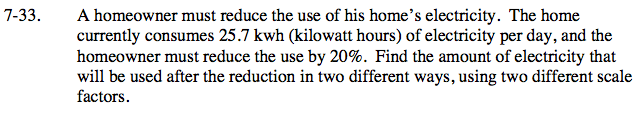Home > CC2MN > Chapter cc27 > Lesson cc27.1.3 > Problem7-33

7-33.

A homeowner must reduce the use of his home’s electricity. The home currently consumes 25.7 kwh (kilowatt hours) of electricity per day, and the homeowner must reduce the use by 20%. Find the amount of electricity that will be used after the reduction in two different ways, using two different scale factors. Homework Help ✎Find 20% of the amount of electricity used.

$\frac{20}{100}=\frac{x}{25.7}$

Once you have found the amount of reduction, subtract that from the original amount of power consumption.

25.7 − 5.14 = 20.56 kwh
If you reduce the use of electricity by 20%, what percentage is left?